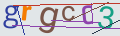# How to change step in input type = number

Changing the step in input type = number is very simple, just use  step attribute:

``<input type="number" name="input_name" step="0.01">``

In this example, clicking on the arrows will switch input in 1/100.

Other example:

``<input type="number" name="input_name" step="0.1">``

You can also specify non-fractions, for example, if you specify step = "2", then input will switch only by even numbers:

``<input type="number" name="input_name" step="2">``

If step attribute is not specified, the default value is 1.

In all those cases user can manually enter any number so if you want to control user entered number you should write a separate validation!